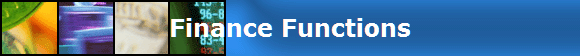FinPak Financial Functions The FinPaK Simulation Functions are not included hereComputes the NPV of a set cash flow using the same convention as the IRR function and also allows for the half-year convention.

Computes the internal rate of return for a series using the half year convention, consistent with NETPV.

Computes the value of an option on a non-dividend paying stock using the Black-Scholes model. Also provides output about the sensitivity of the option

 BSOPMTEXT

Provides handy way to label the output from BSOPM

Computes the value of an option on a dividend paying stock using the Black-Scholes model. Also provides output about the sensitivity of the option

 BSDOPMTEXT

Provides handy way to label the output from BSDOPM

Estimates the implied variance of the rate or return on the underlying security if you have information about a call option and the underlying security. It is a useful way to get the market's estimate of the standard deviation.

This function calculates an option value based on the Binomial Option Pricing Model. Like Black-Scholes it assumes a European Option.

 BIOPMTEXT

Provides handy way to label the output from BIOPM

This function calculates an option value based on the Binomial Option Pricing Model. It might be easier to supply the inputs since the values are in an annual form and it supplies an estimate of the Standard Deviation given the values for the Up and Down States

 BIYOPMTEXT

Provides handy way to label the output from BIYOPM

This function calculates an option value based on the Binomial Option Pricing Model. This version could be called the Binomial Approximation to the Black Scholes model and uses exactly the same inputs. You only need the standard deviation and function will estimate the Up and Down steps

 BIAOPMTEXT

Provides handy way to label the output from BIAOPM[FinPak] [Getting FinPak] [Installation] [Help] [Fin Functions] [Simulation] [The Greeks] [Array Functions] [Root Finding]Copyright 2003 Pieter A. Vandenberg All rights reserved.